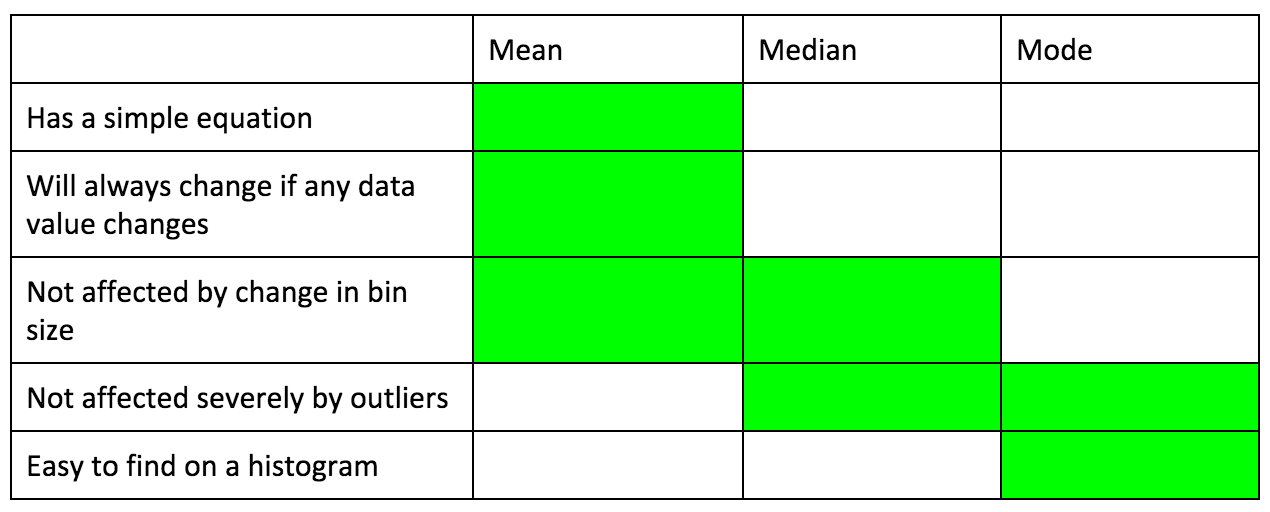PURCHASE A DIGITAL COPY PURCHASE A HARD COPY Lesson 1 Introduction to Statistical Research Methods Lesson 2 Visualizing Data Lesson 3 Central Tendency Lesson 4 Variability Lesson 5 Standardizing Lesson 6 Normal Distribution Lesson 7 Sampling Distributions Lesson 8 Estimation Lesson 9 Hypothesis Testing Lesson 10 t-Tests for Dependent Samples Lesson 11 t-Tests for Independent Samples Lesson 12 Intro to One-Way ANOVA Lesson 13 One-Way ANOVA: Test significance of differences Lesson 14 Correlation Lesson 15 Linear Regression Lesson 16 Chi-Squared Tests Afterward Index

One central idea of statistics is describing groups of numbers with numbers. Mode, mean, and median are three different statistics that can describe groups of numbers in different ways. For example, you can tell that the following three histograms are different, but how would we describe each of them using numbers?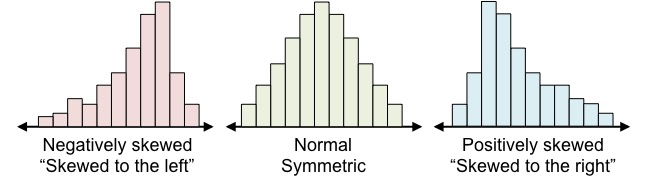Mode
The mode is the value or group of values in a set of numbers that occurs the most. When looking at histograms, which show frequencies in different bins rather than individual values, the mode is the range of values where the frequency is highest. For example, in the following histogram, the mode is the range of values between 6 and 7.If the bin size is too small or too large, the mode is ambiguous. For example, take the following dataset, visualized with a dot plot.

 • • • • • • 1 2 3 4 5 6 7 8 9 10

A histogram of this data with bin size 5 looks like this: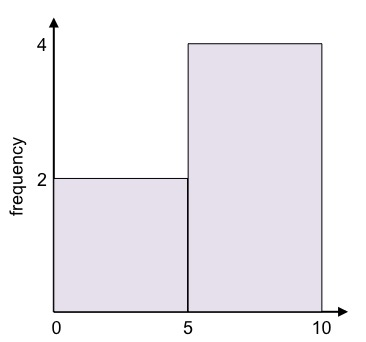Clearly, most of the values are between 5 and 10, so this is the mode. But if we make the bin size 1, the histogram looks like this:Now there is no longer a clear mode. So, it’s important to choose a proper bin size so that you can easily visualize the data.

There are pros and cons to using the mode to describe a dataset. You can probably discover these for yourself by thinking about the following questions:

1. Can the mode be used to describe any kind of data (numerical or categorical)?
2. Do all values in a dataset affect the mode? In other words, if we included an additional value in the dataset or changed an existing value, would the mode always change?
3. If we take many samples from the same population, will the mode be the same in each sample?
4. Can we calculate the mode with an equation?

Mean
The mean is another commonly-used metric to describe data. Think of this number as the fulcrum of a scale.You would probably guess that this scale would tip to the left. So, where should the center beam be placed so that the scale is perfectly balanced? That point is like the mean.

You can also think of it like this: in order for the three differently-sized objects on the left to be equal in weight to the three equally-sized objects on the right, how big should each object on the right be?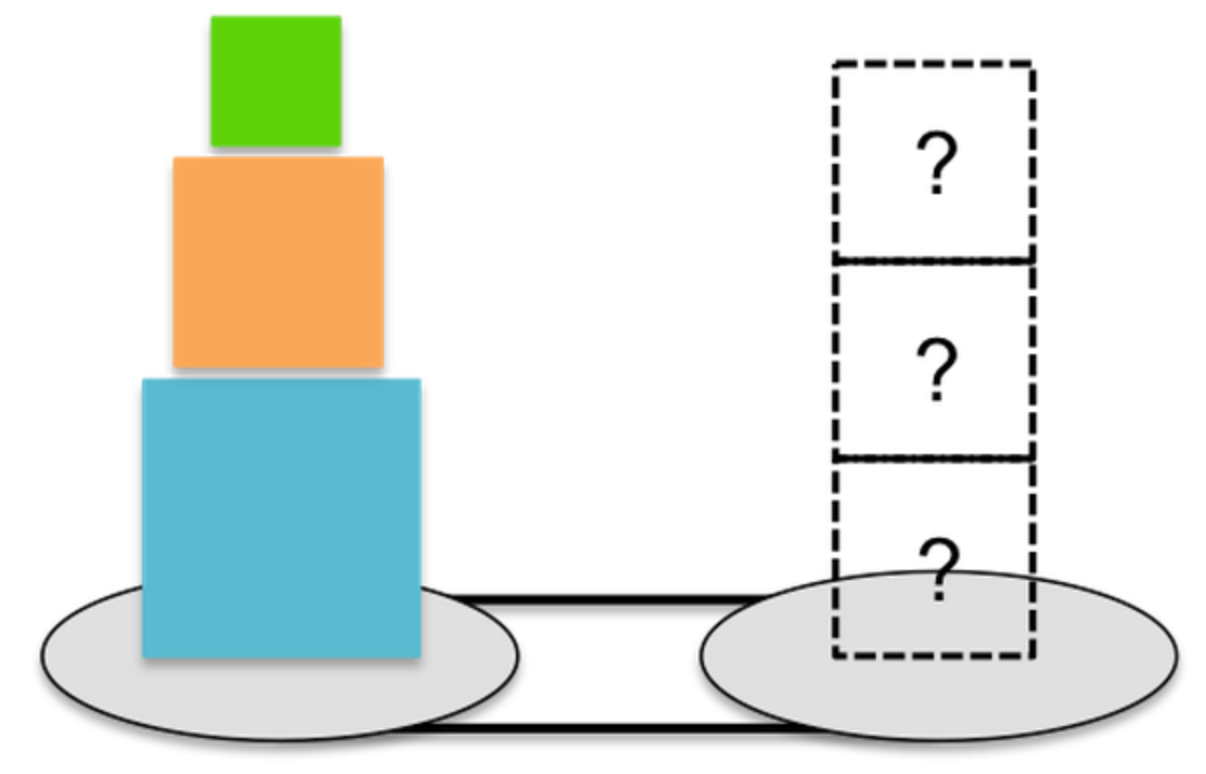Let’s say the green square is size x; the orange square is size y; and the blue square is size z. Let’s say that the unknown sizes on the right are size m, and m is what we’re trying to find out.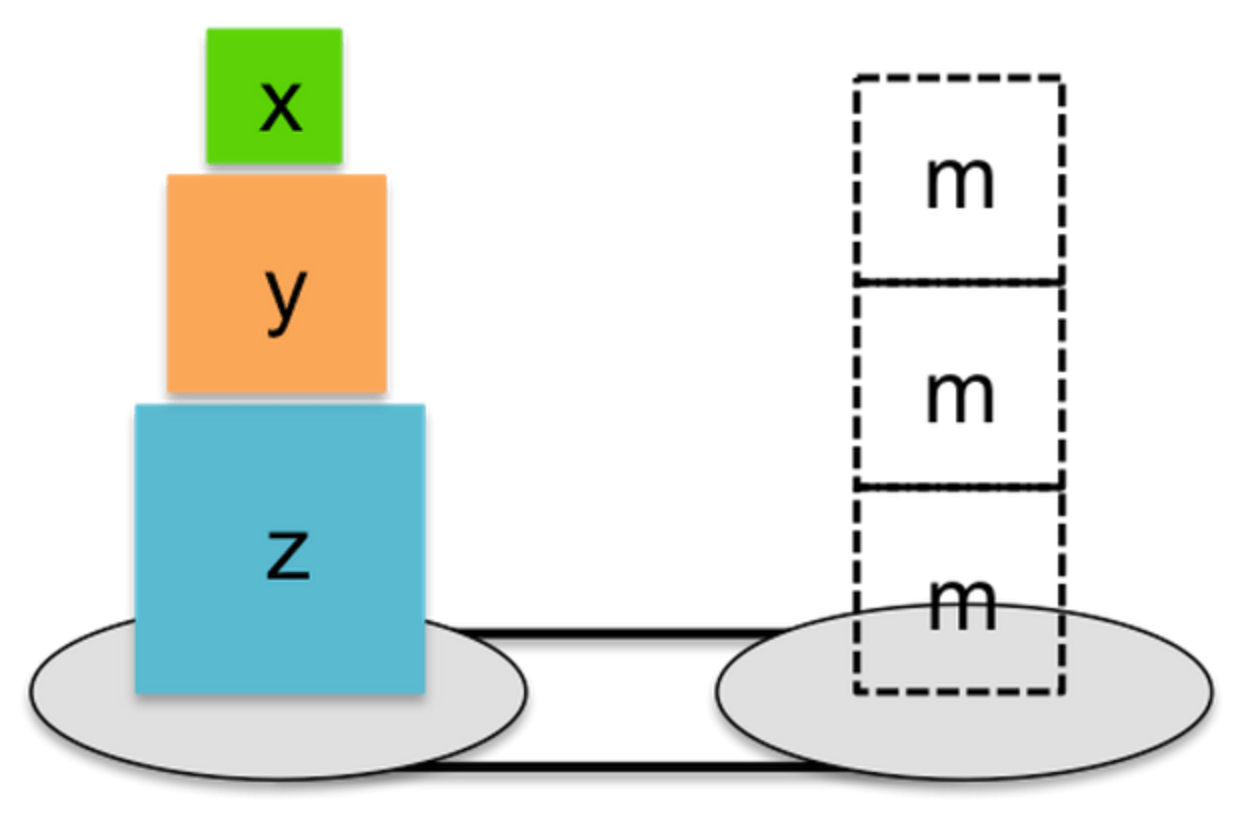We can then say that x + y + z = m + m + m x + y + z = 3m

and therefore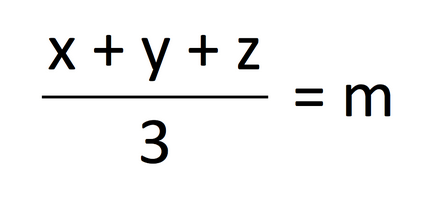where m is the mean.

The mean for a sample is generally symbolized as x̄ (x-bar) while the mean for a population is symbolized as μ (mu). We can more generally symbolize the mean asDon’t be scared by this notation. This is simply a more generic way of saying that the mean is the sum of the values divided by the number of values.

The Greek letter capital sigma (Σ) represents the function of taking the sum of everything that comes after it. You see that “i = 1” is on the bottom and “n” is on the top, meaning we take the sum of x1, x2, x3, all the way to xn. So, we could rewrite these equations as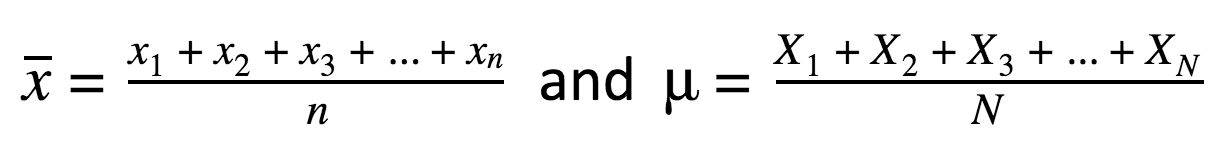R Tutorial: Find the mean Using R to find the mean couldn’t be easier. Let’s practice with the data for starting salaries of geography majors shown in the quiz. Let’s input this data into R and call it “geo.” geo = c(48670, 57320, 38150, 41290, 53160) Now we can simply type mean(geo) Practice using spreadsheets to find the mean Let’s compare the mean of a population with that from several random samples. The following command will generate 500 values that have a mean of 50 and a standard deviation of 4. (Don’t worry about standard deviation too much — this is a measure of how much the data is spread out and you’ll learn about it in Lesson 4.) pop = rnorm(500, 50, 4) Next, find the mean of this “population”: mean(pop) Finally, take a random sample (let’s say size 20) from this population and find the mean. samp = sample(pop, 20) mean(samp) Input these two commands a few more times. You should get values that are scattered close to mean(pop).

One important property about the mean is it’s extremely volatile, meaning that certain values in the dataset can seriously affect the mean. One practical example of this is Michael Jordan, who was a geography major, but whose salary was over \$500,000. Try using R to calculating the mean if we include 500,000 in our “geo” data (maybe call it “geo2”). What happens to the mean?

Therefore, while the mean is less ambiguous than the mode, has a clear calculation, and often summarizes the data well, it doesn’t tell the whole story. This is why we also use the median as a measure of center.

Median
The median is a single value that is greater than half the other values in the dataset and therefore also less than half the values. For example, in the dataset

 8 5 9 13 12

which number is greater than half and less than half? It’s helpful to first put the data in order from least to greatest or greatest to least:

 5 8 9 12 13

Then you see that 9 is greater than two values and less than two values. In the case of a dataset with an odd number of values, one of the actual listed values will be the median.

 4 9 11 13 18 22

Now there are two values in the center: 11 and 13. In the case of an even-numbered dataset, we take the average of the middle two values. For this dataset, the median is 12.

We can also find the approximate location of the median when data is visualized as a histogram.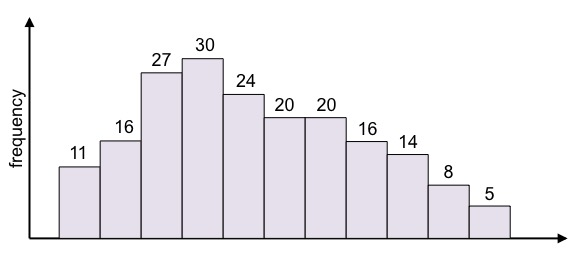In which bin do you think the median would be? Remember that half the values are less than the median, and half the values are greater. How many values are there in total in this dataset? We would add all the frequencies:

11 + 16 + 27 + 30 + 24 + 20 + 20 + 16 + 14 + 8 + 5 = 191

If we put all values in the dataset in order, which value (in which place — the first, second, third, etc.) would be the median? If you think you know, skip to the next paragraph. If you’re unsure, let’s take a step back and think about a dataset with only 3 values. If we put them in order, the median will be the second value. What about a dataset with 5 values? In this case, the median will be the third value. For a dataset with 7 values, the median will be the 4th value. In each case, we added 1 to the total number of values, and then divided by 2.

There is an odd number of values (191), so the median will be the value smack in the middle. In a dataset with 191 values, the median is greater than 95 values and less than 95 values. (95 + 95 = 190 values, and if we include the median we have 191 values.) We add 1 to 191 and divide by 2 to find the place of the median: (191 + 1)/2 = 96.

In the histogram above, where will the 96th value be? We have to add the frequencies until we pass 96. (Numbers in blue are frequencies depicted in the histogram.)

11 + 16 = 27

27 + 27 = 54

54 + 30 = 84

84 + 24 = 108

So, we know that the median is in the orange bin.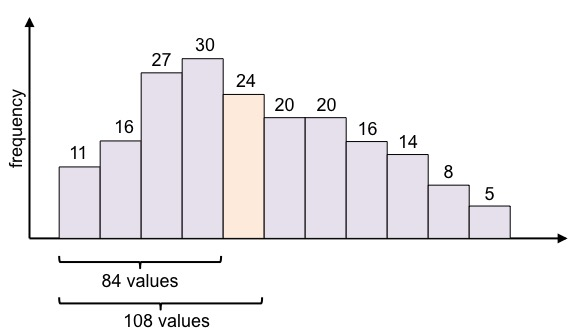What would an outlier do to the median? Let’s use the same two datasets and include an outlier, 80.

 Dataset 1: 5 8 9 12 13 80 Dataset 2: 4 9 11 13 18 22 80

The new median of Dataset 1 is the average of 9 and 12 → 10.5
The new median of Dataset 2 is 13.
You can see that including an outlier did not change the median by much.

Now that you’ve learned about the three measures of center — mode, mean, and median — try the following exercises to dive a little deeper into how they work together to describe the shape of data.

Exercises: Analyze distributions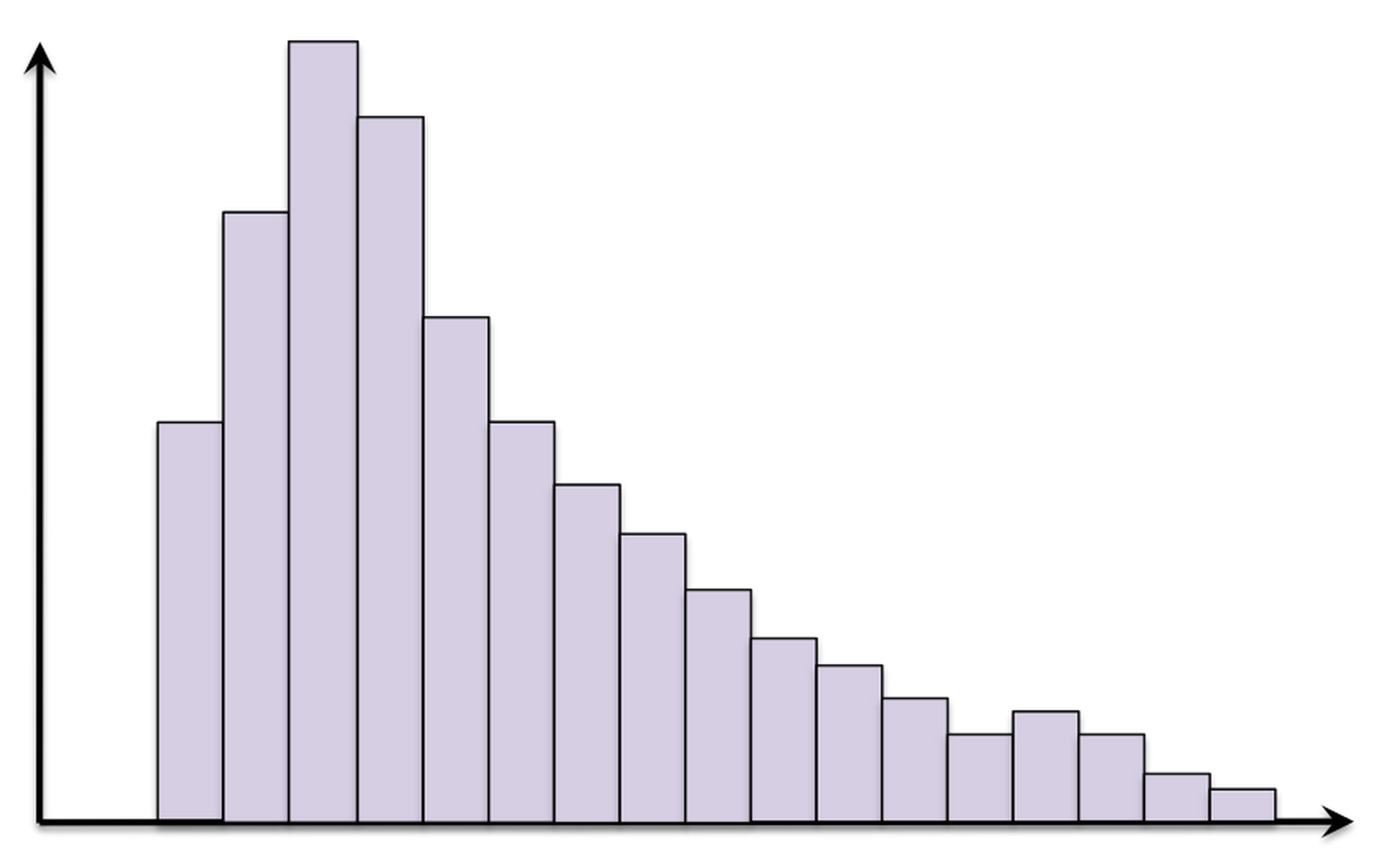• mean < median < mode
• median < mode < mean
• mode < median < mean
• mode < mean < median

2. Which symbols (<, >, =) should go in the blanks to make this statement true for this distribution? [quiz]

mean ___ median ___ mode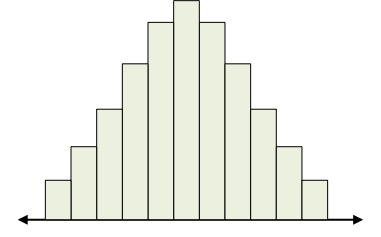3. In the table below, the row headers list positive characteristics that we’ll ideally have in a measure of center. Which characteristics are true for each measure? [quiz]

 Mean Median Mode Has a simple equation Will always change if any data value changes Not affected by change in bin size Not affected severely by outliers Easy to find on a histogram